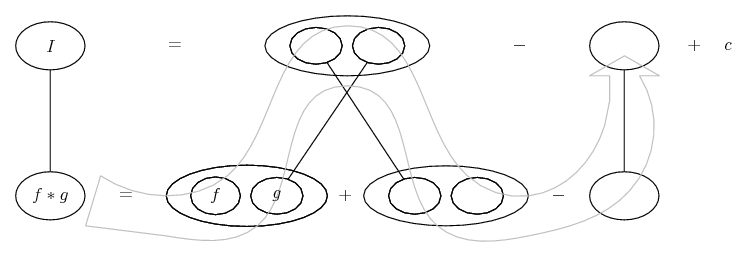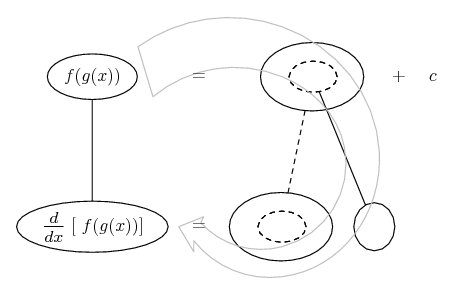# Thread: Integrate LN2x/x^2

1. ## Integrate LN2x/x^2

Hey guys, thanks for all the help so far.. stuck on another problem.

$\displaystyle \int \frac{ln2x}{x^2}$

So I think
$\displaystyle ln2x$
is an integration by parts problem by itself, but I am lost.
I know that
$\displaystyle \int lnx = xlnx-x$
But that was just a rule given by the professor, not sure how we applied that.

I think it goes something like this (using integration by parts)
$\displaystyle u= ln2x \ du= \frac{2x}{x} \ dv=dx \ v=x$

Using the rule
$\displaystyle UV- \int VdU$

I get
$\displaystyle (xln2x)- \int x \frac{2x}{x}$
which equals
$\displaystyle (xln2x)- \int \frac{2x^2}{x}$

But that's just for the top portion of the original and I am not even sure I did that correctly.

Thanks guys

2. Just in case a picture helps...... where (key in first spoiler) ...

Spoiler:... is the product rule. Straight continuous lines differentiate downwards (integrate up) with respect to x. And,... is lazy integration by parts, doing without u and v.

Spoiler:________________________________________

Don't integrate - balloontegrate!

Balloon Calculus; standard integrals, derivatives and methods

Balloon Calculus Drawing with LaTeX and Asymptote!

3.Originally Posted by SpoolxHey guys, thanks for all the help so far.. stuck on another problem.

$\displaystyle \int \frac{ln2x}{x^2}$

So I think
$\displaystyle ln2x$
is an integration by parts problem by itself, but I am lost.
I know that
$\displaystyle \int lnx = xlnx-x$
But that was just a rule given by the professor, not sure how we applied that.

I think it goes something like this (using integration by parts)
$\displaystyle u= ln2x \ du= \frac{2x}{x} \ dv=dx \ v=x$

Using the rule
$\displaystyle UV- \int VdU$

I get
$\displaystyle (xln2x)- \int x \frac{2x}{x}$
which equals
$\displaystyle (xln2x)- \int \frac{2x^2}{x}$

But that's just for the top portion of the original and I am not even sure I did that correctly.

Thanks guys
$\displaystyle ln(2x)=ln2+lnx$

$\displaystyle \displaystyle\int{\frac{ln(2x)}{x^2}}dx=ln2\int{\f rac{1}{x^2}}dx+\int{\frac{lnx}{x^2}}dx$

Now you can use integration by parts.

4. Hello, Spoolx!

$\displaystyle \displaystyle \int \frac{\ln(2x)}{x^2}\,dx$

Integrate by parts:

. . $\displaystyle \begin{array}{cccccccc} u &=& \ln(2x) && dv &=& \dfrac{1}{x^2}\,dx \\ \\[-3mm] du &=& \dfrac{dx}{x} && v &=& \text{-}\dfrac{1}{x} \end{array}$

And we have: .$\displaystyle \displaystyle \text{-}\frac{1}{x}\ln(2x) + \int\frac{dx}{x^2}$

. . . . . . . . $\displaystyle \displaystyle =\;\text{-}\frac{1}{x}\ln(2x) - \frac{1}{x} + C$

. . . . . . . . $\displaystyle \displaystyle =\;\text{-}\frac{1}{x}\left[\ln(2x) + 1\right] + C$

5. Thanks for the help guys, can you jst run through the derivation of the $\displaystyle ln2x$ please?

6. Just in case a picture helps...... where (key in spoiler) ...

Spoiler:... is the chain rule. Straight continuous lines differentiate downwards (integrate up) with respect to the main variable (in this case ), and the straight dashed line similarly but with respect to the dashed balloon expression (the inner function of the composite which is subject to the chain rule).

The general drift is...Though, as Archie Meade points out, you can split the log first to avoid the chain rule.

_________________________________________

Don't integrate - balloontegrate!

Balloon Calculus; standard integrals, derivatives and methods

Balloon Calculus Drawing with LaTeX and Asymptote!

7.Originally Posted by SpoolxHey guys, thanks for all the help so far.. stuck on another problem.

$\displaystyle \int \frac{ln2x}{x^2}$

So I think
$\displaystyle ln2x$
is an integration by parts problem by itself, but I am lost.
I know that
$\displaystyle \int lnx = xlnx-x$
But that was just a rule given by the professor, not sure how we applied that.

I think it goes something like this (using integration by parts)
$\displaystyle u= ln2x \ du= \frac{2x}{x} \ dv=dx \ v=x$

Using the rule
$\displaystyle UV- \int VdU$

I get
$\displaystyle (xln2x)- \int x \frac{2x}{x}$
which equals
$\displaystyle (xln2x)- \int \frac{2x^2}{x}$

But that's just for the top portion of the original and I am not even sure I did that correctly.

Thanks guys
Rather than trying to apply integration by parts just to the numerator,
you should try applying the technique to the entire expression.

$\displaystyle \displaystyle\int{u}dv=uv-\int{v}du$

$\displaystyle u=ln(2x),\;\;dv=\displaystyle\frac{1}{x^2}dx$

We need $\displaystyle v$ and $\displaystyle du$ to apply integration by parts. Using the chain rule we get

$\displaystyle \displaystyle\frac{du}{dx}=\frac{1}{2x}2$

$\displaystyle \Rightarrow\ du=\frac{1}{x}dx$

$\displaystyle v=\int{x^{-2}}dx=-x^{-1}$

$\displaystyle \displaystyle\ uv-\int{v}du}=-\frac{ln(2x)}{x}+\int{\frac{1}{x^2}dx=-\frac{ln(2x)}{x}-\frac{1}{x}+C$

Alternatively, begin with

$\displaystyle ln(2x)=ln2+lnx$

8. Glad I found this forum, learning alot from you guys!
Thanks!